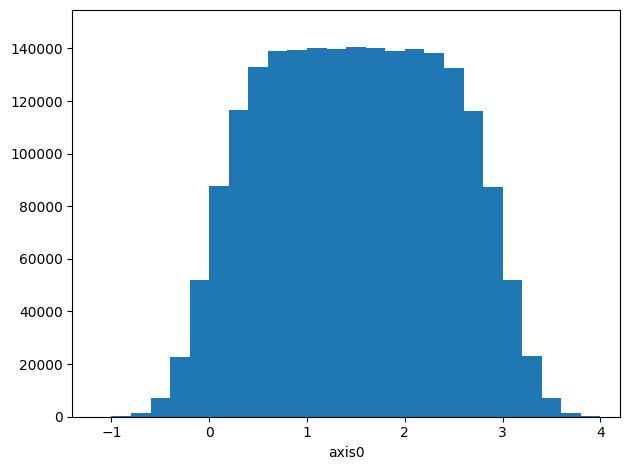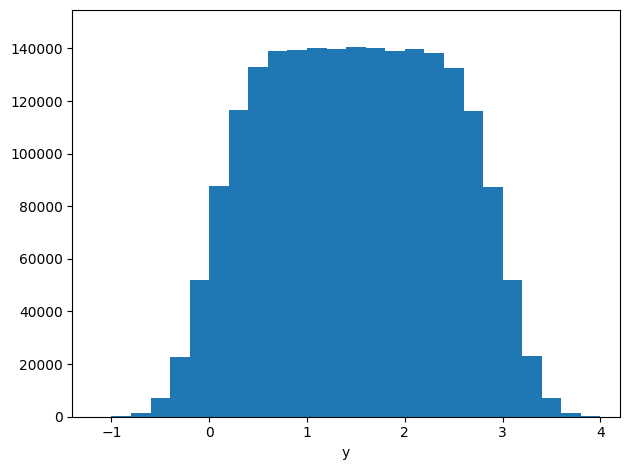It is possible to operate on dask arrays and spare the memory (or perhaps even time).

:
# Necessary imports
import physt
import numpy as np

from physt import h1, h2
%matplotlib inline
:
# Create two arrays
np.random.seed(42)

SIZE = 2 ** 21
CHUNK = int(SIZE / 16)

million = np.random.rand(SIZE)#.astype(int)
million2 = (3 * million + np.random.normal(0., 0.3, SIZE))#.astype(int)

chunked = da.from_array(million, chunks=(CHUNK))
chunked2 = da.from_array(million2, chunks=(CHUNK))

Create histograms¶

h1, h2, … have their alternatives in physt.dask_compat. They should work similarly. Although, they are not complete and unexpected errors may occur.

:
from physt.compat.dask import h1 as d1
from physt.compat.dask import h2 as d2
:
# Use chunks to create a 1D histogram
ha = d1(chunked2, "fixed_width", bin_width=0.2)
check_ha = h1(million2, "fixed_width", bin_width=0.2)
ok = (ha == check_ha)
print("Check: ", ok)
ha.plot()
ha
Check:  True
:
Histogram1D(bins=(28,), total=2097152, dtype=int64):
# Use chunks to create a 2D histogram
hb = d2(chunked, chunked2, "fixed_width", bin_width=.2, axis_names=["x", "y"])
check_hb = h2(million, million2, "fixed_width", bin_width=.2, axis_names=["x", "y"])
hb.plot(show_zero=False, cmap="rainbow")
ok = (hb == check_hb)
print("Check: ", ok)
hb
Check:  True
:
Histogram2D(bins=(5, 28), total=2097152, dtype=int64):
# And another cross-check
hh = hb.projection("y")
hh.plot()
print("Check: ", np.array_equal(hh.frequencies, ha.frequencies))   # Just frequencies
Check:  True:
# Use dask for normal arrays (will automatically split array to chunks)
d1(million2, "fixed_width", bin_width=0.2) == ha
:
True

Some timings¶

Your results may vary substantially. These numbers are just for illustration, on 4-core (8-thread) machine. The real gain comes when we have data that don’t fit into memory.

Efficiency¶

:
# Standard
%time h1(million2, "fixed_width", bin_width=0.2)
Wall time: 361 ms
:
Histogram1D(bins=(28,), total=2097152, dtype=int64)
:
# Same array, but using dask
%time d1(million2, "fixed_width", bin_width=0.2)
Wall time: 116 ms
:
Histogram1D(bins=(28,), total=2097152, dtype=int64)
:
%time d1(chunked2, "fixed_width", bin_width=0.2)
Wall time: 91.8 ms
:
Histogram1D(bins=(28,), total=2097152, dtype=int64)

Different scheduling¶

:
%time d1(chunked2, "fixed_width", bin_width=0.2)
Wall time: 76 ms
:
Histogram1D(bins=(28,), total=2097152, dtype=int64)
:
%%time